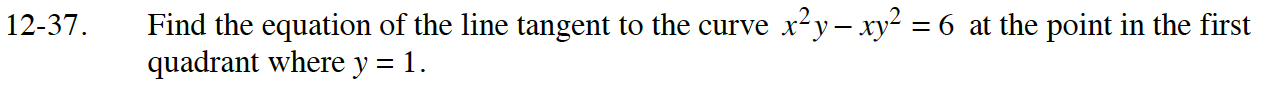### Home > CALC > Chapter Ch12 > Lesson 12.1.3 > Problem12-37

12-37.When y = 1, x2x = 6.
Therefore x = ?

Substitute the values of the coordinates of the point into the equation in Step 2. Then solve for y′.

Use the point from Step 1 and the slope (y′) from Step 3 to write the equation of the tangent line.